### REPT Function Examples in Excel, VBA, & Google Sheets

This Tutorial demonstrates how to use the Excel REPT Function in Excel to repeat text a number of times.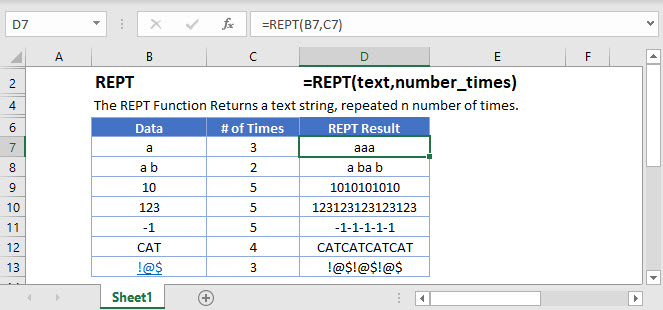## REPT Function Overview

The REPT Function Returns a text string, repeated n number of times.

To use the REPT Excel Worksheet Function, select a cell and type: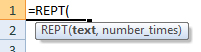(Notice how the formula inputs appear)

## REPT Function Syntax and Inputs:

text – A string of text.

number_times – The number of times to repeat a string a text. If zero, then empty text (“”) Is returned.

AutoMacro - VBA Code Generator

## How to use the REPT Function in Excel:

The REPT function repeats text a given number of times.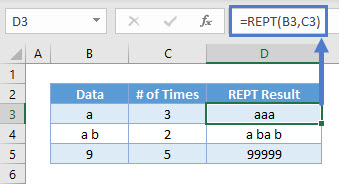As seen above, B3:B5 data is repeated in D3:D5 the number of times shown in C3:C5.

## Decimals or 0

If you enter a decimal value for number_times, REPT will truncate the decimal and take the whole number. If you place a zero, nothing comes up.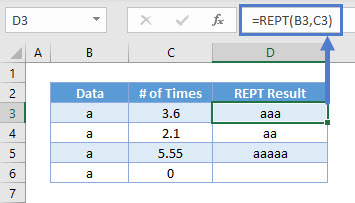## Create in Cell Charts

You can use the REPT Function as a quick alternative to Data Bars to display a mini-chart inside a cell: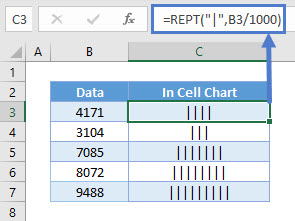REPT repeats the pipe symbol (“|”) a number of times determined by dividing the number by 1000, creating a mini-chart.

## Find Last Text in Range

The REPT Function is also useful (a long with the LOOKUP Function)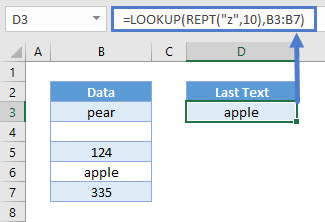Excel works like a dictionary when it comes to determining which text is “largest”. “A” is the smallest, and “Z” is the largest. And within each alphabet, the subsequent alphabet is the next determining factor. For instance, “Za…” is deemed as smaller than “Ze…” since “e” is larger than “a”.

There are no words that repeat “z” 10 times (“zzzzzzzzzz”), so this is safe enough to assume it’s the largest text you can find. Think of LOOKUP as going backwards from cell B7 to B3 to find something smaller or equals to a “zzzzzzzzzz”. It skips cell B7, since that is a number. It goes backwards to cell B6, and it is a text smaller or equals to “zzzzzzzzzz”.

## Split by Common Delimiter

You might want to split all the words within a cell into different rows. To do so, you can use this formula: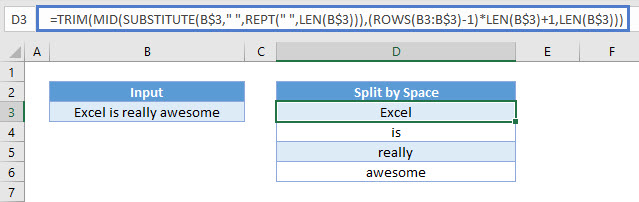This formula uses SUBSTITUTE to substitute all the spaces with a large number of repeated spaces using REPT. It results in the text looking like this:
“Excel                       is                       really                       awesome”

The number of repeated spaces equals the length of the entire string. Using this information, we can extract each word by using the MID Function to extract the entire length of the original string. This allows us to capture each word within the MID Function, but nothing else, leaving only the original word after the TRIM Function is applied.

The ROWS Function allows us to easily specify each word number as the formula is copied down.

## REPT Function in Google Sheets

The REPT Function works exactly the same in Google Sheets as in Excel: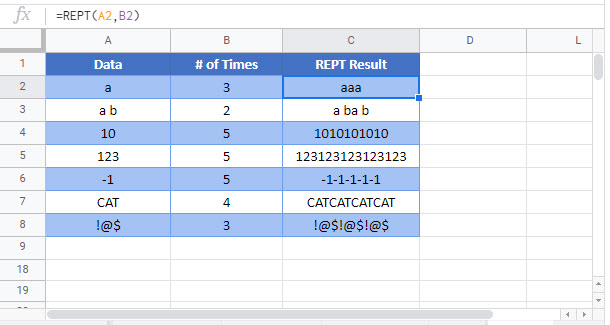## REPT Examples in VBA

You can also use the REPT function in VBA. Type:
`application.worksheetfunction.rept(text,number_times)`

Executing the following VBA statements

will produce the following results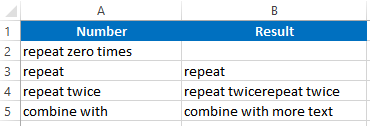For the function arguments (text, etc.), you can either enter them directly into the function, or define variables to use instead.

Return to the List of all Functions in Excel

## Excel Practice Worksheet

Practice Excel functions and formulas with our 100% free practice worksheets!

• Automatically Graded Exercises
• Learn Excel, Inside Excel!